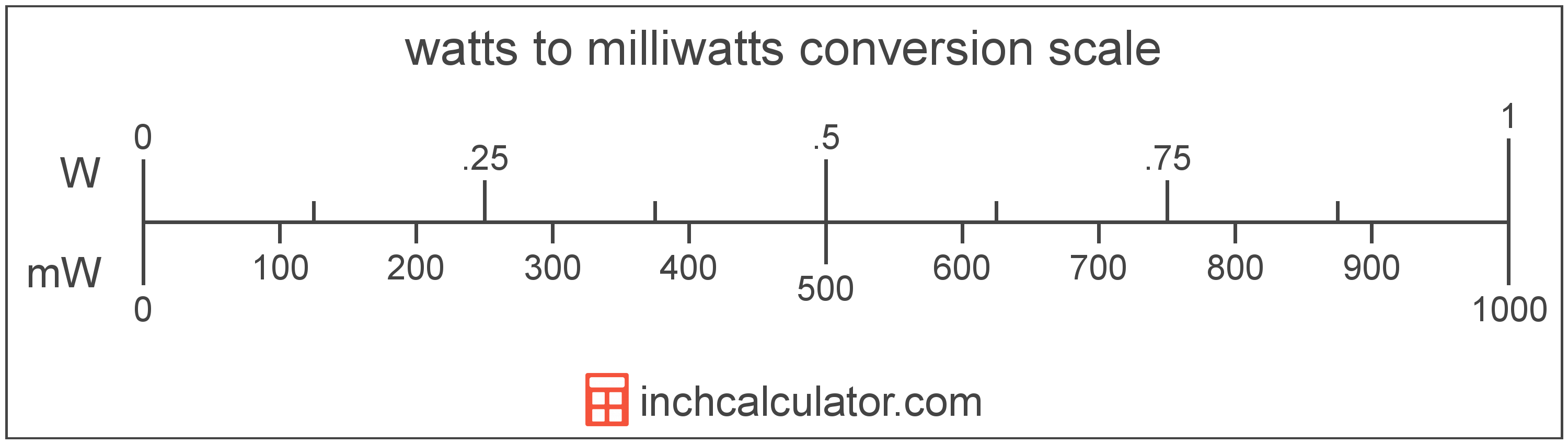# Milliwatts to Watts Conversion

Enter the power in milliwatts below to get the value converted to watts.

Results in Watts:1,000,000 mW = 1,000 W
Do you want to convert watts to milliwatts?

## How to Convert Milliwatts to WattsTo convert a milliwatt measurement to a watt measurement, divide the power by the conversion ratio.

Since one watt is equal to 1,000 milliwatts, you can use this simple formula to convert:

watts = milliwatts ÷ 1,000

The power in watts is equal to the milliwatts divided by 1,000.

For example, here's how to convert 5,000 milliwatts to watts using the formula above.
5,000 mW = (5,000 ÷ 1,000) = 5 W

## Milliwatts

One milliwatt is the power equal to 1/1,000 of a watt, or the energy consumption at a rate of 1/1,000 of a joule per second.

The milliwatt is a multiple of the watt, which is the SI derived unit for power. In the metric system, "milli" is the prefix for 10-3. Milliwatts can be abbreviated as mW; for example, 1 milliwatt can be written as 1 mW.

## Watts

One watt is the power equal to the rate of energy of one joule per second in an electrical circuit. One watt is also equal to the power produced in a circuit with a voltage potential of one volt with a current of one amp.

The watt is the SI derived unit for power in the metric system. Watts can be abbreviated as W; for example, 1 watt can be written as 1 W.

Watt's Law states the relationship between power, current, and voltage. Using Watt's Law, it's possible to express the power in watts as an expression using current and voltage.

PW = IA × VV

The power in watts is equal to the current in amperes times the potential difference in volts.

Combining this with Ohm's Law, it's also possible to express the power in watts using resistance in addition to volts and amps.

## Milliwatt to Watt Conversion Table

Milliwatt measurements converted to watts
Milliwatts Watts
1 mW 0.001 W
2 mW 0.002 W
3 mW 0.003 W
4 mW 0.004 W
5 mW 0.005 W
6 mW 0.006 W
7 mW 0.007 W
8 mW 0.008 W
9 mW 0.009 W
10 mW 0.01 W
20 mW 0.02 W
30 mW 0.03 W
40 mW 0.04 W
50 mW 0.05 W
60 mW 0.06 W
70 mW 0.07 W
80 mW 0.08 W
90 mW 0.09 W
100 mW 0.1 W
200 mW 0.2 W
300 mW 0.3 W
400 mW 0.4 W
500 mW 0.5 W
600 mW 0.6 W
700 mW 0.7 W
800 mW 0.8 W
900 mW 0.9 W
1,000 mW 1 W

## References

1. International Bureau of Weights and Measures, The International System of Units, 9th Edition, 2019, https://www.bipm.org/documents/20126/41483022/SI-Brochure-9-EN.pdf